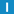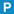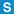# class LinearHashTable

Library: Foundation
Package: Hashing

## Description

This class implements a linear hash table.

In a linear hash table, the available address space grows or shrinks dynamically. A linear hash table thus supports any number of insertions or deletions without lookup or insertion performance deterioration.

Linear hashing was discovered by Witold Litwin in 1980 and described in the paper LINEAR HASHING: A NEW TOOL FOR FILE AND TABLE ADDRESSING.

The LinearHashTable is not thread safe.

Value must support comparison for equality.

Find, insert and delete operations are basically O(1) with regard to the total number of elements in the table, and O(N) with regard to the number of elements in the bucket where the element is stored. On average, every bucket stores one element; the exact number depends on the quality of the hash function. In most cases, the maximum number of elements in a bucket should not exceed 3.

## Member Summary

Member Functions: begin, bucketAddress, bucketAddressForHash, buckets, calcSize, clear, count, empty, end, erase, find, insert, merge, operator =, size, split, swap

## Types

### Bucket

typedef std::vector < Value > Bucket;

### BucketIterator

typedef typename Bucket::iterator BucketIterator;

### BucketVec

typedef std::vector < Bucket > BucketVec;

### BucketVecIterator

typedef typename BucketVec::iterator BucketVecIterator;

### ConstPointer

typedef const Value * ConstPointer;

### ConstReference

typedef const Value & ConstReference;

### Hash

typedef HashFunc Hash;

### Pointer

typedef Value * Pointer;

### Reference

typedef Value & Reference;

### ValueType

typedef Value ValueType;

## Constructors

### LinearHashTableLinearHashTable(
std::size_t initialReserve = 64
);

Creates the LinearHashTable, using the given initialReserve.

### LinearHashTableLinearHashTable(
const LinearHashTable & table
);

Creates the LinearHashTable by copying another one.

## Destructor

### ~LinearHashTable~LinearHashTable();

Destroys the LinearHashTable.

## Member Functions

### beginConstIterator begin() const;

Returns an iterator pointing to the first entry, if one exists.

### beginIterator begin();

Returns an iterator pointing to the first entry, if one exists.

### bucketsstd::size_t buckets() const;

Returns the number of allocated buckets.

### clearvoid clear();

Erases all elements.

### countstd::size_t count(
const Value & value
) const;

Returns the number of elements with the given value, with is either 1 or 0.

### emptybool empty() const;

Returns true iff the table is empty.

### endConstIterator end() const;

Returns an iterator pointing to the end of the table.

### endIterator end();

Returns an iterator pointing to the end of the table.

### erasevoid erase(
Iterator it
);

Erases the element pointed to by it.

### erasevoid erase(
const Value & value
);

Erases the element with the given value, if it exists.

### findConstIterator find(
const Value & value
) const;

Finds an entry in the table.

### findIterator find(
const Value & value
);

Finds an entry in the table.

### insertstd::pair < Iterator, bool > insert(
const Value & value
);

Inserts an element into the table.

If the element already exists in the table, a pair(iterator, false) with iterator pointing to the existing element is returned. Otherwise, the element is inserted an a pair(iterator, true) with iterator pointing to the new element is returned.

### operator =LinearHashTable & operator = (
const LinearHashTable & table
);

Assigns another LinearHashTable.

### sizestd::size_t size() const;

Returns the number of elements in the table.

### swapvoid swap(
LinearHashTable & table
);

Swaps the LinearHashTable with another one.

### bucketAddressconst Value & value
) const;

### bucketAddressForHashstd::size_t hash
);

### calcSizestatic std::size_t calcSize(
std::size_t initialSize
);

void merge();

void split();